Question

PROBLEM 6 120 N/m 60 N.m D For the beam and loading shown, (a) draw the...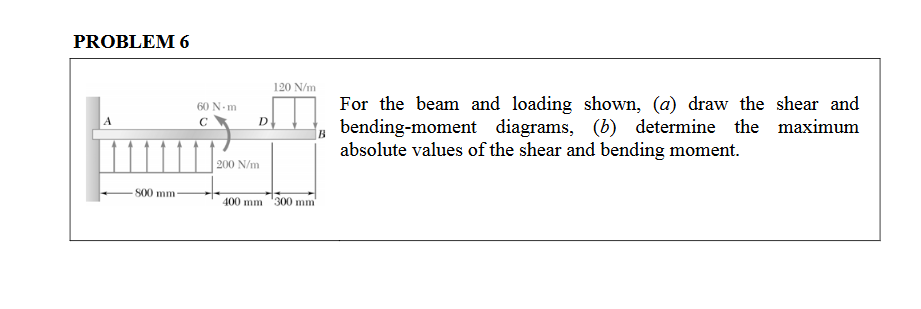PROBLEM 6 120 N/m 60 N.m D For the beam and loading shown, (a) draw the shear and bending-moment diagrams, (b) determine the maximum absolute values of the shear and bending moment. 200 N/m 500 mm 400 mm 300 mm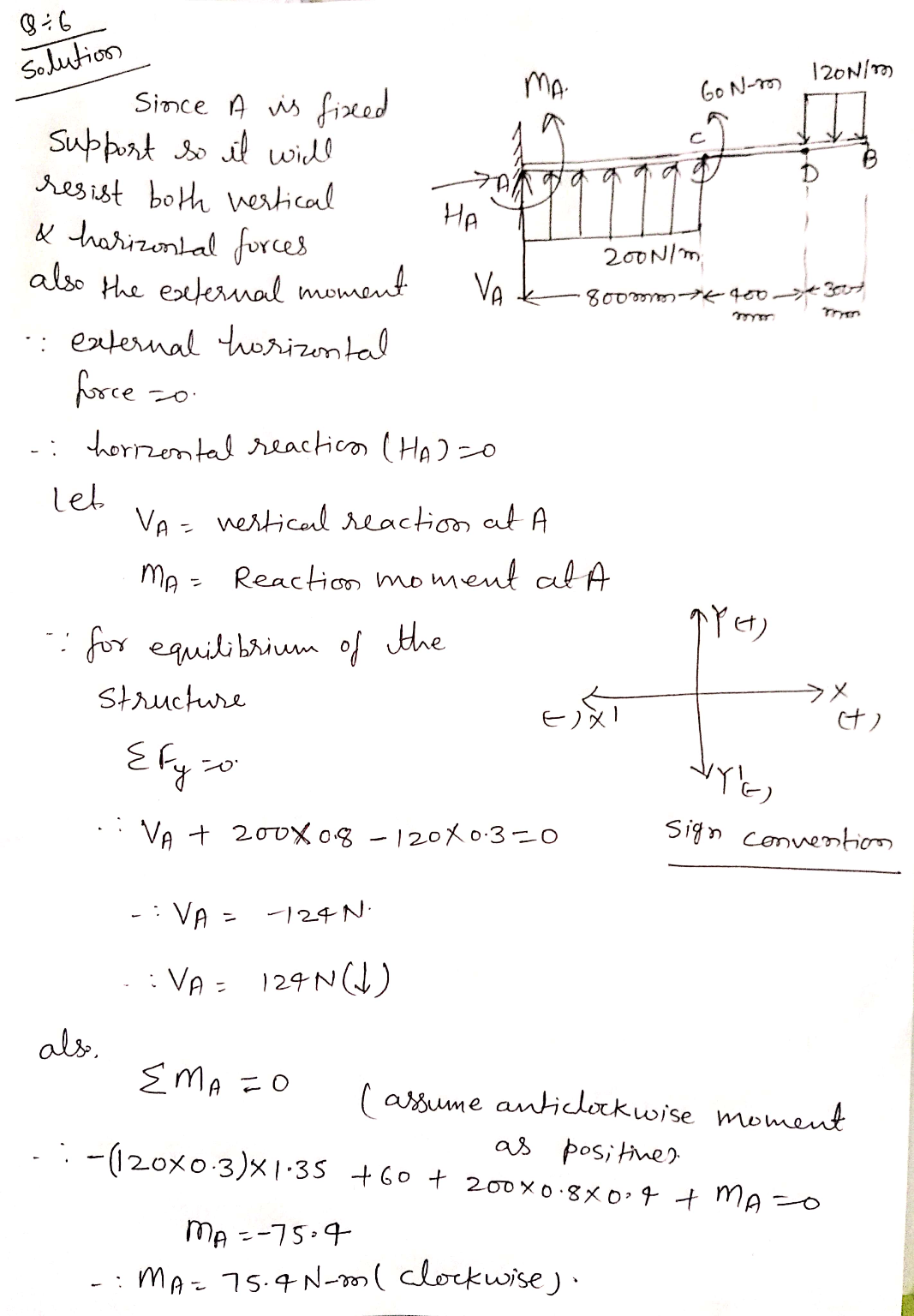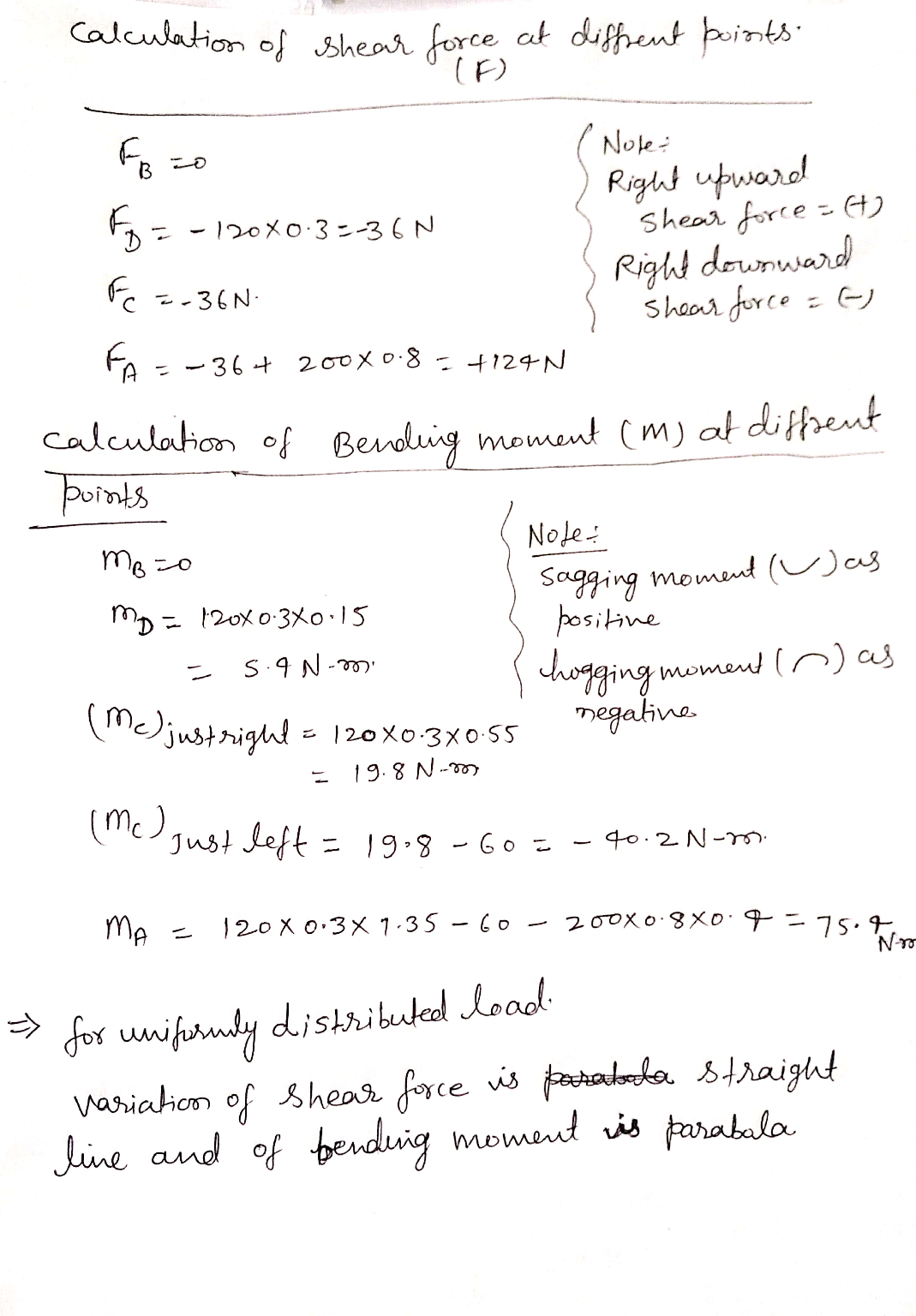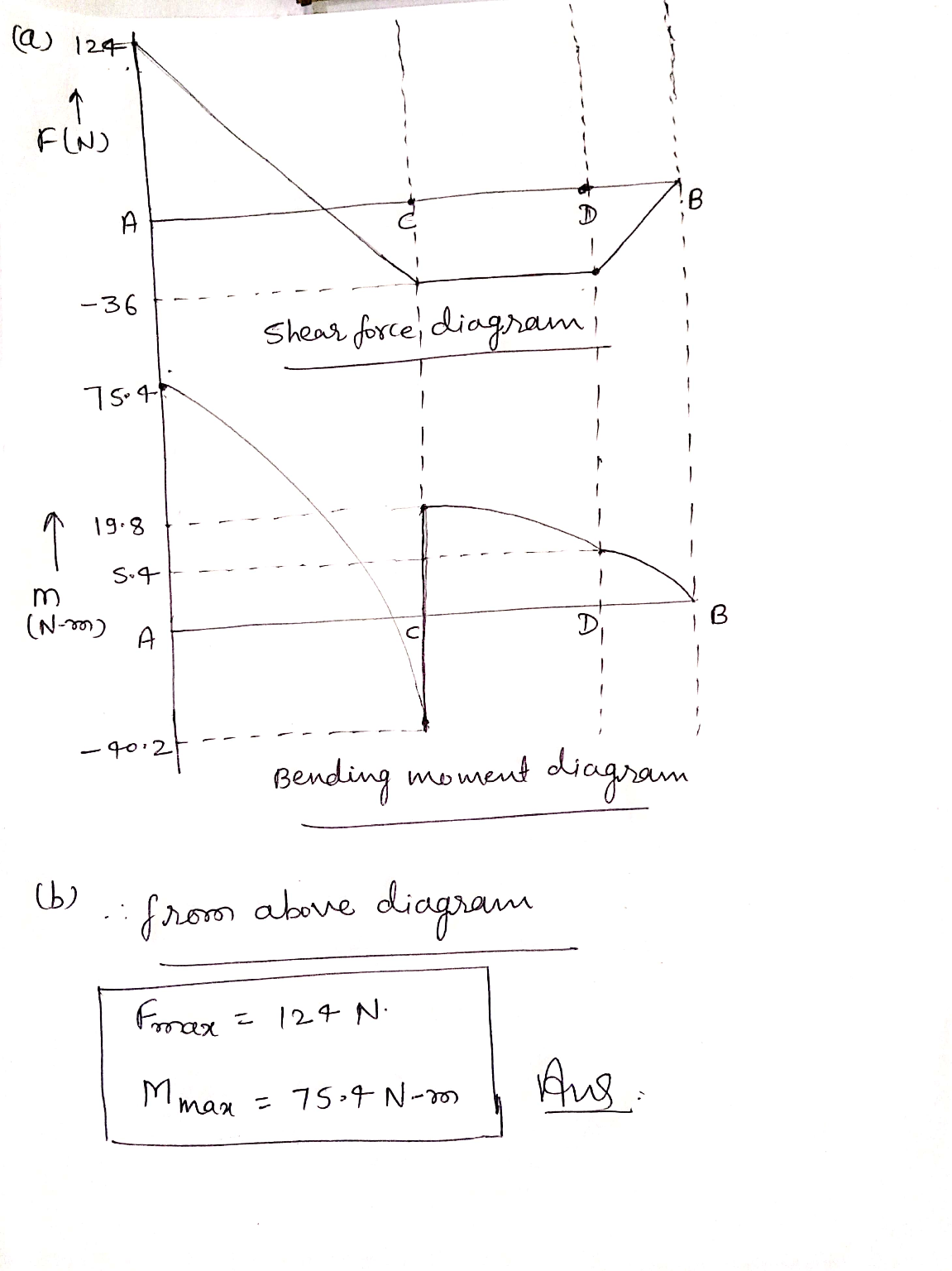Earn Coins

Coins can be redeemed for fabulous gifts.

Similar Homework Help Questions
• PROBLEM 6 120 Nm 50 N с D For the beam and loading shown, (a) draw...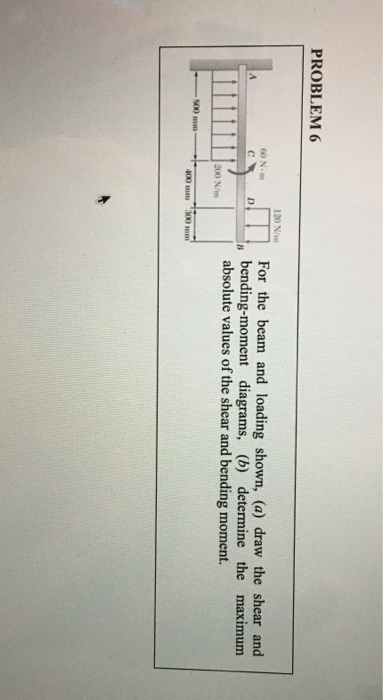PROBLEM 6 120 Nm 50 N с D For the beam and loading shown, (a) draw the shear and bending-moment diagrams, (b) determine the maximum absolute values of the shear and bending moment. 200 N/m 400 mm 300 mm

• PROBLEM 6 120 Nm 50 N с D For the beam and loading shown, (a) draw...PROBLEM 6 120 Nm 50 N с D For the beam and loading shown, (a) draw the shear and bending-moment diagrams, (b) determine the maximum absolute values of the shear and bending moment. 200 N/m 400 mm 300 mm

• Please show your work for a positive rating. 120 N/m 60 Nm D For the beam...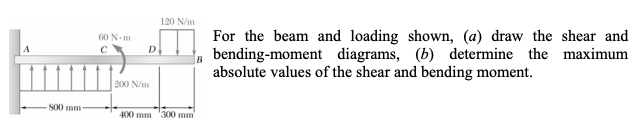Please show your work for a positive rating. 120 N/m 60 Nm D For the beam and loading shown, (a) draw the shear and bending-moment diagrams, (b) determine the maximum absolute values of the shear and bending moment. B 200 N/m 500 mm 400 mm 300 mm

• For the beam and loading shown, (a) draw the shear and bending-moment diagrams, (b) determine the...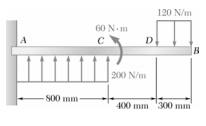For the beam and loading shown, (a) draw the shear and bending-moment diagrams, (b) determine the maximum absolute values of the shear and bending moment. 120 N/m 60 Nm с A D B 200 N/m 500 mm 400 mm 300 mm

• PROBLEM 6 For the beam and loading shown, (a) draw the shear and bending-moment diagrams, (b)...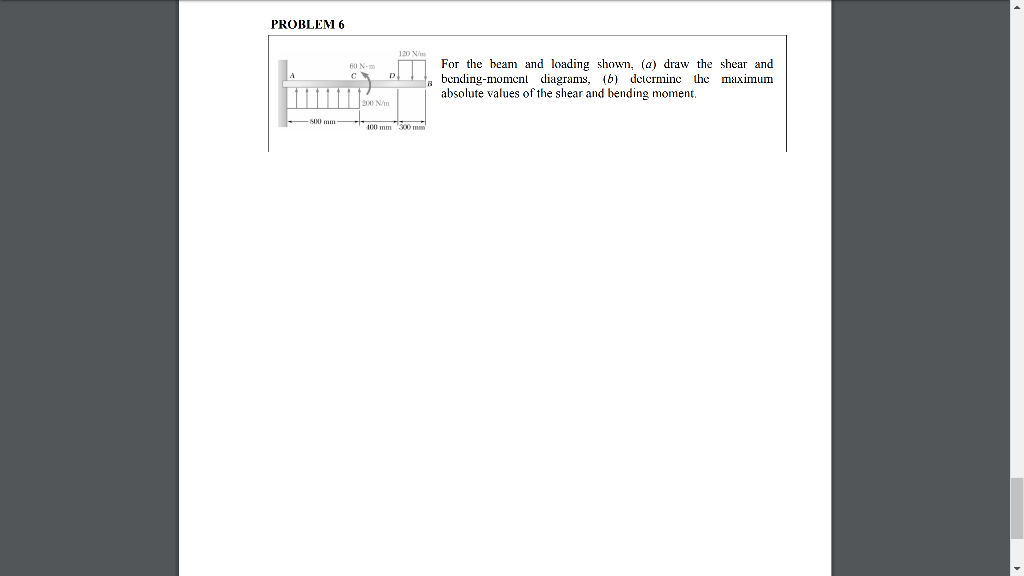PROBLEM 6 For the beam and loading shown, (a) draw the shear and bending-moment diagrams, (b) determine the maximum absolute values of the shear and bending moment. PROBLEM 6 120 W BON For the beam and loading shown, (a) draw the shear and bending-moment diagrams, (6) detcrminc the maximum absolute values of the shear and bending moment, 90 mm 100 mm

• For the beam and loading shown draw the shear and bending moment diagrams and then find...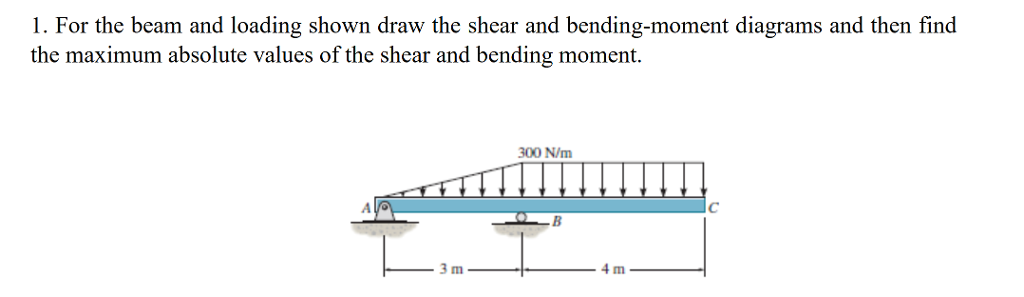For the beam and loading shown draw the shear and bending moment diagrams and then find the maximum absolute values of the shear and bending moment. 1. For the beam and loading shown draw the shear and bending-moment diagrams and then find the maximum absolute values of the shear and bending moment. 300 N/m 4 m

• Problem 1A,B: Draw the shear and bending moment diagrams for the beam and loading shown, and...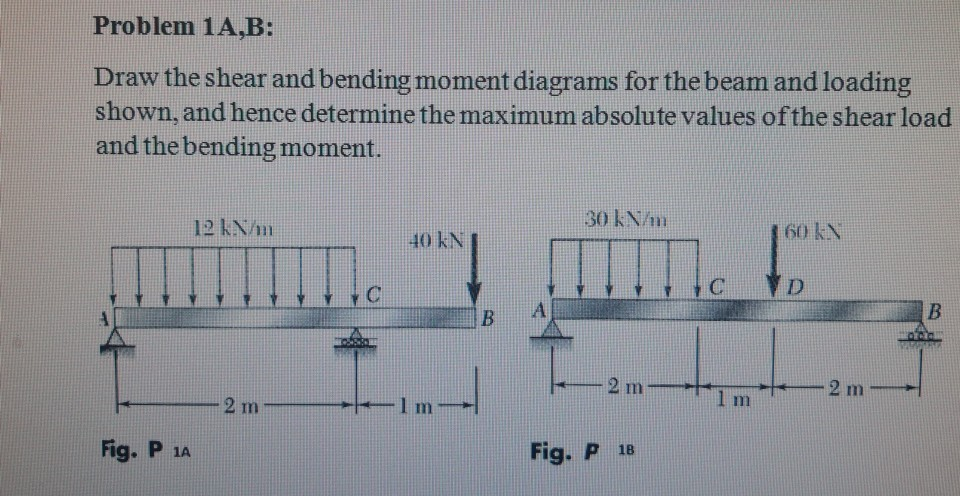Problem 1A,B: Draw the shear and bending moment diagrams for the beam and loading shown, and hence determine the maximum absolute values of the shear load and the bending moment. 30 kN/m 12 km 160 kN D 2 m 2 m 1 m 2 mm Fig. P 12 Fig. P 18

• PROBLEM 7.42 For the beam and loading shown, (a) draw the shear and bending-moment diagrams, (b)...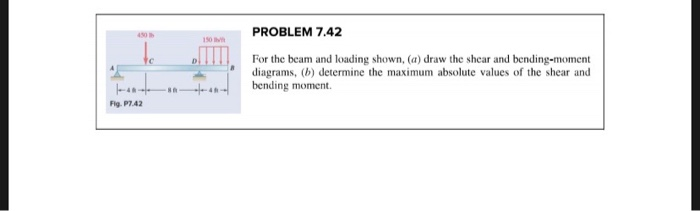PROBLEM 7.42 For the beam and loading shown, (a) draw the shear and bending-moment diagrams, (b) determine the maximum absolute values of the shear and bending moment. - -- -- - Fig. P7.42

• HW7 80 KN PROBLEM 7.40 30 IN For the beam and loading shown, (a) draw the...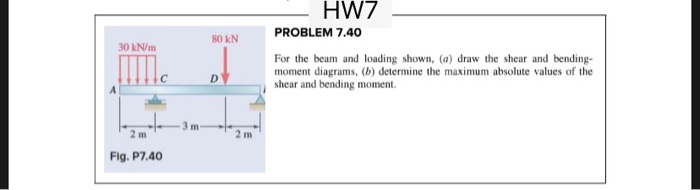HW7 80 KN PROBLEM 7.40 30 IN For the beam and loading shown, (a) draw the shear and bending- moment diagrams, (b) determine the maximum absolute values of the shear and bending moment. Fig. P7.40

• post only Group#7 Problem 5 (6 marks) For the beam and loading shown, a) Draw the...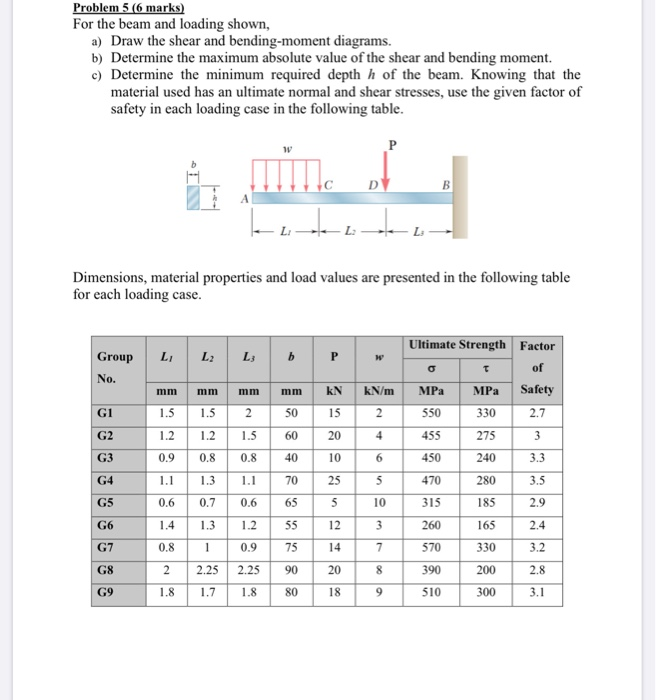post only Group#7 Problem 5 (6 marks) For the beam and loading shown, a) Draw the shear and bending-moment diagrams. b) Determine the maximum absolute value of the shear and bending moment. c) Determine the minimum required depth h of the beam. Knowing that the material used has an ultimate normal and shear stresses, use the given factor of safety in each loading case in the following table. P A LLL Dimensions, material properties and load values are presented in...